# SSAT Middle Level Math : How to find a ratio

## Example Questions

1 2 5 6 7 8 9 10 11 13 Next →

### Example Question #121 : How To Find A Ratio

What is the simplest form of the following ratio: 325:50?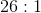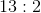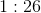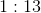Explanation:

In order to determine the simplest form of a ratio, divide both sides of the ratio by a common factor. If we divide each side of this ratio by 5, we get 65:10.

This can still be simplified by dividing by 5 again.

Therefore, the simplest form of the ratio is 13:2.

### Example Question #5 : Use Ratio Reasoning To Convert Measurement Units: Ccss.Math.Content.6.Rp.A.3d

A carpenter is making a model house and he buys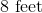of crown molding to use as accent pieces. He needs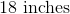of the molding for the house. How many feet of the material does he need to finish the model?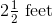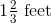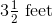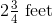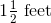Explanation:

We can solve this problem using ratios. There are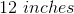in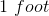. We can write this relationship as the following ratio: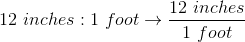We know that the carpenter needs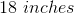of material to finish the house. We can write this as a ratio using the variableto substitute the amount of feet.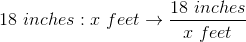Now, we can solve forby creating a proportion using our two ratios.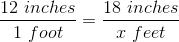Cross multiply and solve for.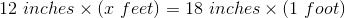Simplify.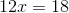Divide both sides by.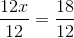Solve.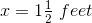The carpenter needs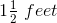of material.

### Example Question #1 : Solving Word Problems With One Unit Conversion

A carpenter is making a model house and he buys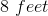of crown moulding to use as accent pieces. He needs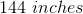of the moulding for the house. How many additional feet of the material will he need to purchase to finish the model?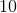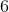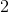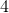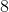Explanation:

We can solve this problem using ratios. There arein. We can write this relationship as the following ratio:We know that the carpenter needsof material to finish the house. We can write this as a ratio using the variableto substitute the amount of feet.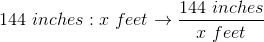Now, we can solve forby creating a proportion using our two ratios.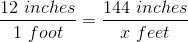Cross multiply and solve for.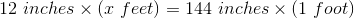Simplify.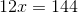Divide both sides by.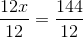Solve.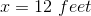The carpenter needs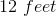of material. Since he already has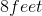he will need to purchasemore to finish the project.

1 2 5 6 7 8 9 10 11 13 Next →

### All SSAT Middle Level Math Resources